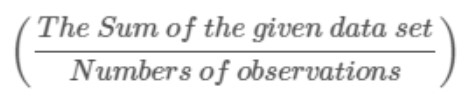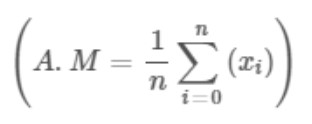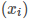# Arithmetic Mean Calculator

To find the arithmetic mean, input the required comma-separated population or sample data set using this arithmetic mean/average calculator.

Give Us Feedback

## Arithmetic Mean calculator

Arithmetic mean calculator is used to calculate the average of the given data set. This arithmetic average calculator calculates the mean of the sample and population data. It provides the step-by-step solution of the given sample or population data.

## How to use this arithmetic mean calculator?

Follow the below steps to calculate the arithmetic mean of sample or population data.

• Select the option first i.e., sample or population.
• Input the comma-separated values.
• Click the calculate button.
• Click the show more button to view the solution with steps.
• Press the reset button to calculate another value.

## Arithmetic mean definition

"The simplest and most frequently used measure of a mean or average is known as the arithmetic mean."

It simply takes the sum of all the given numbers, then divides that sum by the count of the numbers used in the given data set.

## Arithmetic mean formula

The general equation of this type of central tendency is given below.

Arithmetic mean formula =It is usually denoted by x bar (x̅).

x̅ = Σx/n

The general equation of the arithmetic mean can also be written in the form of summation.• A.M is the arithmetic mean.
• n is the total number of observations.
•are the values of the given data set

## How to calculate the arithmetic mean?

Here is a solved example of an arithmetic mean.

Example

Find the arithmetic mean of 1, 21, 43, 12, 14.

Solution

Step 1: Write the given data in ascending order.

1, 12, 14, 21, 43

Step 2: Write the general formula of the arithmetic mean.Step 3: Put the given data values in the formula.

Arithmetic mean = (1 + 12 + 14 + 21 + 43)/5
Arithmetic mean = 91/5
Arithmetic mean = 18.2

## FAQs

### what is the arithmetic mean of 9 and 900?

Answer: The arithmetic mean of 9 and 900 is 454.5

### What is the arithmetic mean of 5 and 125?

Answer: The arithmetic mean of 5 and 125 is 65

### What is the arithmetic mean of 9 and 7?

Answer: The arithmetic mean of 9 and 7 is 8

### What is the arithmetic mean of 4 and 9?

Answer: The arithmetic mean of 4 and 9 is 6.5

### what is the arithmetic mean of 7 and 63?

Answer: The arithmetic mean of 7 and 63 is 35

### Math Tools